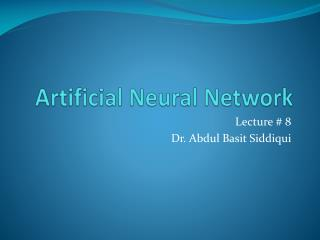DownloadDownload PresentationArtificial Neural Network

# Artificial Neural Network

Download Presentation## Artificial Neural Network

- - - - - - - - - - - - - - - - - - - - - - - - - - - E N D - - - - - - - - - - - - - - - - - - - - - - - - - - -
##### Presentation Transcript

1. Artificial Neural Network Lecture # 8 Dr. Abdul Basit Siddiqui

2. Covered so for (Revision) • 1943 McCulloch and Pitts proposed the McCulloch-Pitts neuron model. • 1949 Hebb published his book The Organization of Behavior, in which the Hebbianlearning rule was proposed. • 1958 Rosenblatt introduced the simple single layer networks now called Perceptrons. • 1969 Minsky and Papert’s book Perceptrons demonstrated the limitation of single layer perceptrons, and almost the whole field went into hibernation. • 1982 Hopfield published a series of papers on Hopfield networks.

3. Covered so for (Revision) • 1982 Kohonen developed the Self-Organising Maps that now bear his name • 1986 The Back-Propagation learning algorithm for Multi-Layer Perceptrons was rediscovered and the whole field took off again. • 1990s The sub-field of Radial Basis Function Networks was developed. • 2000s The power of Ensembles of Neural Networks and Support Vector Machiness becomes apparent.

4. Covered so for (Revision) • Introduction to Biological Neurons and its components • The McCullock-Pitts Neurons • Vastly simplified model of real neurons (Threshold Logic Unit)

5. Covered so for (Revision) • Activation functions • Step Function (Threshold or sign function) • Sigmoid Function

6. Covered so for (Revision) • McCulloch-Pitts Neuron Equation in terms of input and output can be written as: • Θis threshold used to squash neuron’s output

7. Networks of McCulloch-Pitts Neuron • We may have many neurons labeled by indices k, i, j and activation flows between them via synapses with strengths wki , wij:

8. The Perceptron • Any number of McCulloch-Pitts neurons can be connected together in any way. • An arrangement of one input layer of McCulloch-Pitts neurons feeding forward to one output layer of McCulloch-Pitts neurons is known as a Perceptron.

9. Implementing Logic Gates using McCulloch-Pitts Neurons • Logical operators AND, OR and NOT can be implemented using MP neurons. We can easily find it with inception.

10. Need to find Weights Analytically • XOR function • Required to calculate the suitable parameters instead of finding solution by trial and error. • It is required to calculate the weights and thresholds.

11. Finding Weights Analytical for the AND Network • We have two weights w1 and w2 and the threshold Θ, and for each training pattern we need to satisfy • We have four inequalities • There are infinite number of solutions for AND, OR and NOT networks.

12. Perceptron’s Limitations • XOR function • What is problem?? • We need more complex networks • Or we need different activation/threshold/transfer functions • We need to learn parameters

13. Structures of ANN • ANN is a weighted directed graph considering the activation flowing between the processing units through one way connections. • Three types of ANNs • Single-Layer Feed-forward NNs • One input layer, one output layer with no feedback connections (a simple perceptron) • Multi-Layer Feed-forward NNs • One input layer, one output layer, one or more hidden layers with no feedback connections (A multilayer perceptron) • Recurrent NNs • The network has atleast one feedback connection. May or may not have hidden units (A simple Recurrent Network)

14. Network’s Structures Examples

15. More Activation Functions • Sigmoid Functions • These are smooth (differentiable) and monotonically increasing.

16. Bias- A special kind of Weight (Instead of Θ) • To simplify mathematics, consider threshold as a weight such that • Suppose then • Hence the equation of perceptron is simplified as

17. Problems with Perceptron • How to classify the data points using perceptron: • Without calculating large no. of inequalities, we have to find weights and thresholds.

18. Decision Boundaries in Two Dimensions • For simple problems, our perceptron is forming decision boundaries between the classes. • The decision boundary (between out = 0 and out = 1) is at w1in1 + w2in2 - Θ = 0 Along the line • In two dimensions, the boundaries are always straight lines.